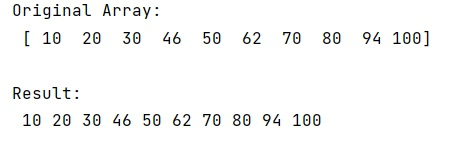# How to print a NumPy array without brackets?

Learn, how to print a NumPy array without brackets in Python?
Submitted by Pranit Sharma, on February 21, 2023

NumPy is an abbreviated form of Numerical Python. It is used for different types of scientific operations in python. Numpy is a vast library in python which is used for almost every kind of scientific or mathematical operation. It is itself an array which is a collection of various methods and functions for processing the arrays.

## Printing a NumPy array without brackets

Suppose that we are given a numpy array that contains some numerical values. When we print a numpy array, the compiler will display the output as an array where all the elements are encapsulated in square brackets [ ]. We need to find a way so we can print a numpy array without brackets.

For this purpose, we need to convert each element of the numpy array into a string and then we will join each string using the str.join() method.

The string is a group of characters, these characters may consist of all the lower case, upper case, and special characters present on the keyboard of a computer system. A string is a data type and the number of characters in a string is known as the length of the string.

Let us understand with the help of an example,

## Python code to print a NumPy array without brackets

```# Import numpy
import numpy as np

# Creating a numpy array
arr = np.array([10,20,30,46,50,62,70,80,94,100])

# Display original array
print("Original Array:\n",arr,"\n")

# Converting each element of array into string
res = map(str, arr)

# Joining the string
res = ' '.join(res)

# Display result
print("Result:\n",res)
```

Output: